Many people know that the sum of the interior angles of a triangle is 180°. So why does the sum of the interior angles of a triangle equal 180°?

Also, triangles are not the only shapes. There are quadrilaterals, pentagons, hexagons, and countless other shapes. These are called polygons. So, what is the sum of the interior angles of a polygon? By understanding this, you will be able to calculate each angle.

After learning about interior angles, we also need to understand exterior angles. By learning the properties of exterior angles, you will be able to find the angles of polygons.

So we will explain how to calculate the sum of interior angles and the sum of exterior angles of polygons, including triangles, quadrilaterals, and pentagons.

## Proof Sum of Interior Angles of a Triangle Is 180°

One of the most important shapes is a triangle. In a triangle, the sum of the interior angles is 180°. Why does the sum of all the interior angles of a triangle add up to 180°? Let’s try to prove this.

In order to prove that the sum of the interior angles is 180°, we need to understand corresponding angles and alternate angles. When two lines are parallel, the corresponding angles are equal. And when two lines are parallel, the alternate angles are equal. By using this property, we can prove that the sum of the interior angles of a triangle is 180°.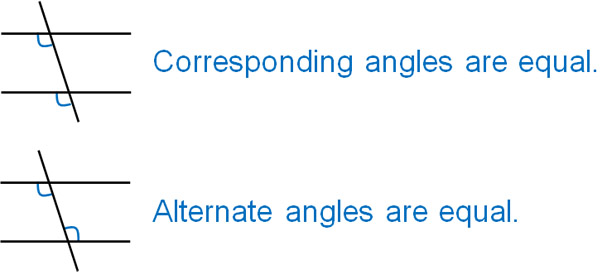First, draw a parallel line to the triangle, as shown below.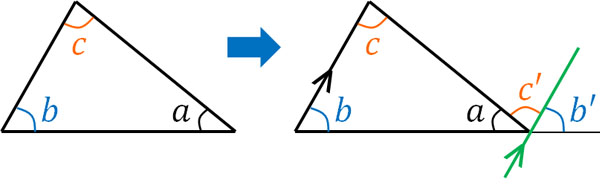Then, what will happen? Corresponding angles are equal, so ∠b and ∠b’ are the same. Also, since the alternate angles are equal, the angles of ∠c and ∠c’ are the same.

So, if we add up ∠a, ∠b, and ∠c, we get a straight line, as shown in the figure. The angle of the straight line is 180°. Therefore, the sum of the interior angles of a triangle is 180°. Thus, we have proved why the sum of the interior angles of the triangle is 180°.

### Relation Between Interior and Exterior Angles of a Triangle

Earlier, we proved that the sum of the interior angles of a triangle is 180°. By proving this, we can see that an exterior angle and the sum of non-adjacent interior angles are equal. In other words, we have the following.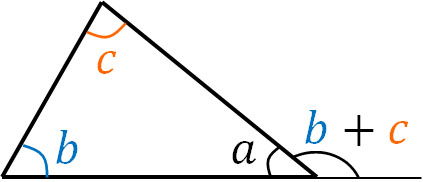So we know that if we want to calculate the exterior angle of a triangle, we just need to sum the interior angles. If we want to calculate the exterior angle of ∠a, we can do the following.

• Exterior angle of ∠a = ∠b + ∠c

Using this method, we can find the exterior angle of ∠a without calculating ∠a. For example, we have the following.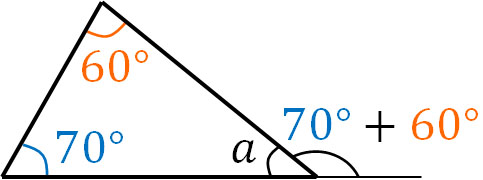Of course, we can calculate the interior angle and then the exterior angle. For example, we can calculate the exterior angle of ∠a is 130° as follows.

• ∠a = 180 – (70 + 60) = 50
• Exterior angle of ∠a = 180 – 50 = 130

However, this method requires more equations. Of course, there will be many miscalculations. Therefore, it is easier to sum the two interior angles to get the exterior angle of ∠a.

## Sum of the Interior Angles of a Quadrilateral or Pentagon

What should we think of shapes with multiple sides, such as quadrilaterals and pentagons, instead of triangles? How much is the sum of the interior angles?

The sum of the interior angles of a polygon increases as follows.

• Triangle: The sum of the interior angles is 180°.
• Quadrilateral: The sum of the interior angles is 360°.
• Pentagon: The sum of the interior angles is 540°.
• Hexagon: The sum of the interior angles is 720°.

As you can see, for each additional side of the polygon, the sum of the interior angles increases by 180°. Using this rule, we can calculate the angles of a polygon.

### Reason Why Sum of Interior Angles Increases by 180° for Each Additional Side

Why does the sum of the interior angles increase by 180° as the number of sides increases by one? Let’s understand the reason for this. To understand this, all we need to know is that the sum of the interior angles of a triangle is 180°.

As an example, let’s consider the sum of the interior angles of a quadrilateral. If we draw a diagonal line on a quadrilateral, we get two triangles.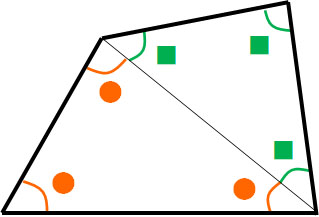Naturally, if we add all the interior angles of the two triangles, we get the sum of the interior angles of the quadrilateral. The sum of the interior angles of a quadrilateral is the sum of all the numbers ● and ■.

And as we already know, the sum of a triangle is 180°. So, if you add all the ●, you get 180°. If you add all the ■, you get 180°. Therefore, we see that the sum of the interior angles of a quadrilateral is 360°.

Similarly, for pentagons and hexagons, draw diagonals to form triangles as shown below.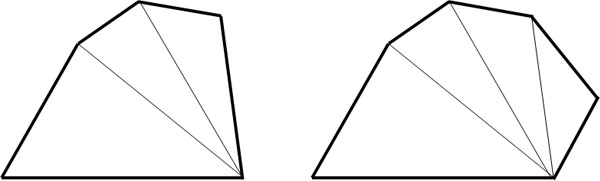The pentagon has three triangles. Therefore, the total is 540°. The hexagon has four triangles. So the total is 720°. Using the properties of triangles, we can understand why they increase by 180°.

For polygons, you may forget how much the sum of the interior angles is. In such cases, think about how many triangles you can make by drawing diagonals. You can then multiply the number of triangles by 180 to get the sum of the interior angles.

### The Sum of All Exterior Angles of a Polygon Is 360°

So far, we have discussed the sum of the interior angles of a polygon. On the other hand, what is the sum of the exterior angles of a polygon? Understand the sum of the exterior angles of triangles, quadrilaterals, and pentagons. Note that the exterior angles are the following parts.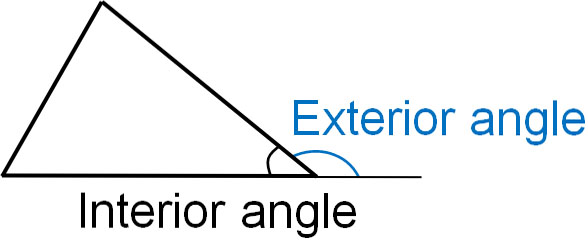For the sum of the exterior angles, it is 360° for all polygons. Triangles, quadrilaterals, and pentagons all have exterior angles that sum to 360°.

Why do all polygons have exterior angles that sum to 360°? Let’s think about the reason. In order to prove this reason, we need to create a formula for the sum of the interior angles of a polygon. To review, it is as follows.

• Triangle: The sum of the interior angles is 180°.
• Quadrilateral: The sum of the interior angles is 360°.
• Pentagon: The sum of the interior angles is 540°.
• Hexagon: The sum of the interior angles is 720°.

From this fact, we can see that the sum of the interior angles of a polygon with n sides has the following formula.

• Sum of the interior angles: $(n-2)×180$

Also, for a polygon, if we add one interior angle and one exterior angle, we get 180° as follows.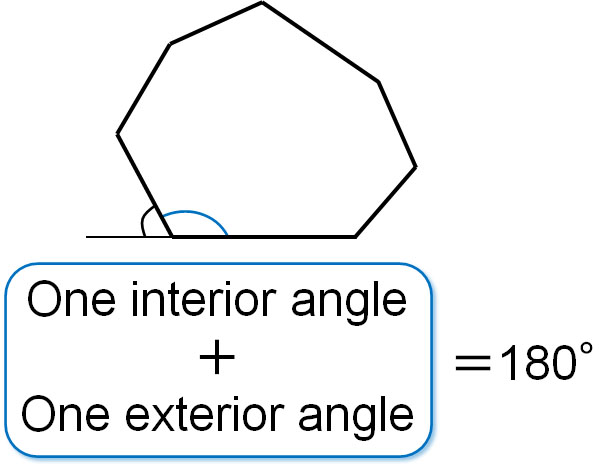For a polygon with n sides, we know that the sum of all the interior and exterior angles is $180×n$. So let’s subtract the sum of the interior angles from the sum of the interior and exterior angles. The result is as follows.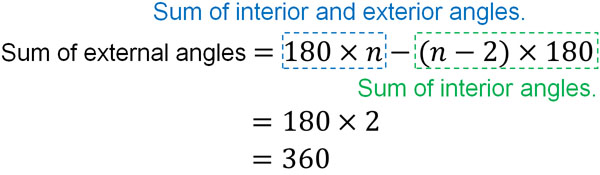Thus, we have proved that for all polygons, the sum of the exterior angles is always 360°. While the sum of the interior angles varies, the sum of the exterior angles is always 360°.

## Exercises: Calculating the Angles of a Polygon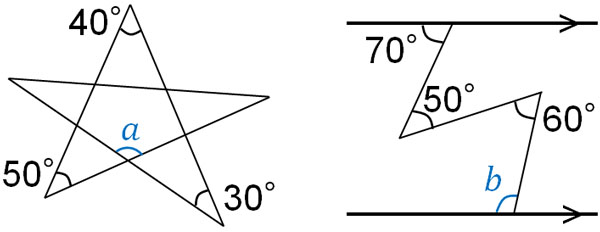(a)

If you know that the sum of the interior angles of a triangle is 180°, you can find ∠a. There are two ways to find the answer. One is to focus on the triangle below.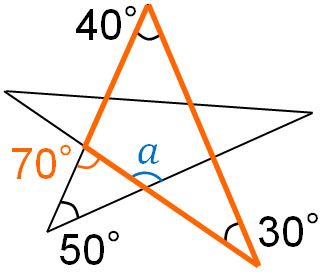We have already explained that if we add two interior angles, we get an exterior angle. Therefore, for the orange angle, the angle will be 70°, as follows.

• $40+30=70$

Also, ∠a is an exterior angle of the triangle. Therefore, using the angle we just calculated, we can calculate that ∠a is 120° as follows.

• $∠a=70+50=120$

For reference, you can also calculate the angle by focusing on another triangle. Focus on the following triangle.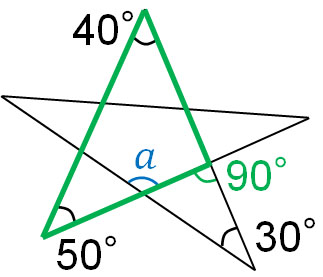In this case, the green angle (the exterior angle of the triangle) is 90°. Therefore, ∠a is 120°, as shown below.

• $∠a=90+30=120$

The answer will be the same. It doesn’t matter which method you use; just use the one that is easier to calculate.

(b)

In many cases, it is not possible to answer a question about a figure using only the problem sentence. In such cases, try to draw a line. If you do not draw a line, it is often impossible to answer the question. For this problem, draw a line as follows.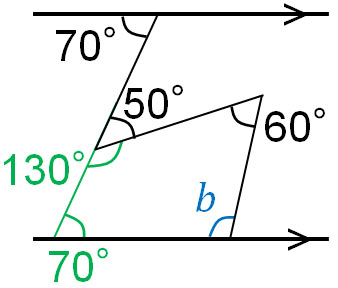In this way, you will have a quadrilateral. For this quadrilateral, write each angle as shown in the above figure. Then you can find the angles of the quadrilateral except for ∠b. The sum of the interior angles of the quadrilateral is 360°. Therefore, ∠b is 100°.

• $∠b=360-(70+130+60)=100$

In angle problems, you should write angles you find on the figure. Also, draw various lines and write the angles. As a result, you will be able to get the answer at some point.

## Properties of Interior and Exterior Angles of Polygons

The sum of the interior angles of a triangle is 180°. However, few people understand why it is 180°, so let’s understand the reason beforehand. By proving that the sum of the interior angles of a triangle is 180°, you will understand that adding two interior angles makes an exterior angle.

Also, the sum of the interior angles of a polygon increases by 180° as the number of sides increases by one. As the number of sides increases, the sum of the interior angles also increases. On the other hand, the sum of the exterior angles is always 360°.

Use these properties to solve geometry problems. For angle problems, write down the angles that you can find using the provided information. In some cases, it is also important to draw lines. This is the key to solving the problem.

If you understand the properties of figures, you will be able to solve angle problems. Understand the angle properties of polygons, not only triangles but also quadrilaterals, pentagons, and other polygons.